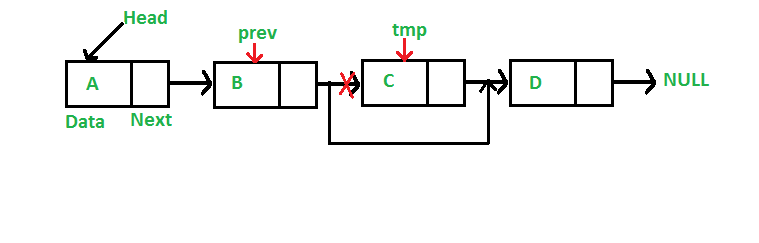# Java Program For Deleting A Node In A Linked List

• Last Updated : 15 Jun, 2022

We have discussed Linked List Introduction and Linked List Insertion in previous posts on a singly linked list.
Let us formulate the problem statement to understand the deletion process. Given a ‘key’, delete the first occurrence of this key in the linked list

Iterative Method:
To delete a node from the linked list, we need to do the following steps.
1) Find the previous node of the node to be deleted.
2) Change the next of the previous node.
3) Free memory for the node to be deleted.Since every node of the linked list is dynamically allocated using malloc() in C, we need to call free() for freeing memory allocated for the node to be deleted.

## Java

 `// A complete working Java program``// to demonstrate deletion``// in singly linked list``class` `LinkedList {``    ``Node head; ``// head of list` `    ``/* Linked list Node*/``    ``class` `Node {``        ``int` `data;``        ``Node next;``        ``Node(``int` `d)``        ``{``            ``data = d;``            ``next = ``null``;``        ``}``    ``}` `    ``/* Given a key, deletes the first``       ``occurrence of key in``     ``* linked list */``    ``void` `deleteNode(``int` `key)``    ``{``        ``// Store head node``        ``Node temp = head, prev = ``null``;` `        ``// If head node itself holds the key to be deleted``        ``if` `(temp != ``null` `&& temp.data == key) {``            ``head = temp.next; ``// Changed head``            ``return``;``        ``}` `        ``// Search for the key to be deleted, keep track of``        ``// the previous node as we need to change temp.next``        ``while` `(temp != ``null` `&& temp.data != key) {``            ``prev = temp;``            ``temp = temp.next;``        ``}` `        ``// If key was not present in linked list``        ``if` `(temp == ``null``)``            ``return``;` `        ``// Unlink the node from linked list``        ``prev.next = temp.next;``    ``}` `    ``/* Inserts a new Node at front of the list. */``    ``public` `void` `push(``int` `new_data)``    ``{``        ``Node new_node = ``new` `Node(new_data);``        ``new_node.next = head;``        ``head = new_node;``    ``}` `    ``/* This function prints contents of linked list starting``       ``from the given node */``    ``public` `void` `printList()``    ``{``        ``Node tnode = head;``        ``while` `(tnode != ``null``) {``            ``System.out.print(tnode.data + ``" "``);``            ``tnode = tnode.next;``        ``}``    ``}` `    ``/* Driver program to test above functions. Ideally this``    ``function should be in a separate user class. It is kept``    ``here to keep code compact */``    ``public` `static` `void` `main(String[] args)``    ``{``        ``LinkedList llist = ``new` `LinkedList();` `        ``llist.push(``7``);``        ``llist.push(``1``);``        ``llist.push(``3``);``        ``llist.push(``2``);` `        ``System.out.println("``Created Linked list is:");``        ``llist.printList();` `        ``llist.deleteNode(``1``); ``// Delete node with data 1` `        ``System.out.println(``            ``"``Linked List after Deletion of ``1``:");``        ``llist.printList();``    ``}``}`

Output:

```Created Linked List:
2  3  1  7
Linked List after Deletion of 1:
2  3  7```

Time Complexity: O(n), where n represents the length of the given linked list.
Auxiliary Space: O(1), no extra space is required, so it is a constant.

Please refer complete article on Linked List | Set 3 (Deleting a node) for more details!

My Personal Notes arrow_drop_up